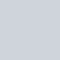# 25 or More – Lit Vapes Mix & Match

In Stock

1 Review

From: \$520.78

Select at least 25 vapes down below and enjoy crazy discounts

• Quantity
• Quantity
• Quantity
• Quantity
• Quantity
• Quantity
• Quantity
• Quantity
• Quantity
• Quantity
• Quantity
• Quantity
• Quantity
• Quantity
• Quantity
• Quantity
• Quantity
• Quantity
• Quantity
• Quantity
• Quantity
• Quantity
• Quantity
• Quantity
• Quantity
• Quantity
• Quantity
• Quantity
• Quantity
• Quantity
• Quantity

## 1 review for 25 or More – Lit Vapes Mix & Match

1.Terrence Sumida

Waiting to try